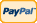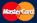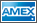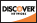•Electronics, electrical engineering
•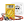Engineering physics
•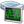Generators
•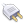Interfaces
•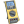Multimeters
•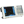Oscilloscopes
•Persona
•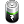Power supplies
•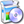Software
•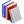Standards
•Thesaurus
•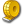Units of measurement
Filter by first letter
A
B
C
D
E
F
G
H
I
J
K
L
M
N
O
P
Q
R
S
T
U
V
W
X
Y
Z
+
-

# Interpolation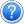Interpolation in digital storage oscilloscopes is continuous signal reconstruction on a limited reports number (samples).

Oppositely to analogue oscilloscopes DSOs don’t measure the input signal continuously but in discrete time only. To display an oscillogram the input signal is restored by its discrete values what is considered the interpolation task of the continuous function on its finite number of points. In DSO interpolation is the mathematical treatment of the input digitalized signal by the device software tools. Depending on the mathematical methods used for the interpolation there can be several interpolation types as follows:

• linear interpolation,
• polynomial interpolation (Bessel or Akima splines)
• (Sin x)/x interpolation  etc.

Different interpolation types are shown in the pictures (sine signal with the frequency of 24MHh is sent to the oscilloscope input).

At the sample rate (recording) of the device of 100MHz for the one signal period there are 4-5 samples what fails to form the displayed oscillogram as sinus.

Linear interpolation for this example is shown in the picture below.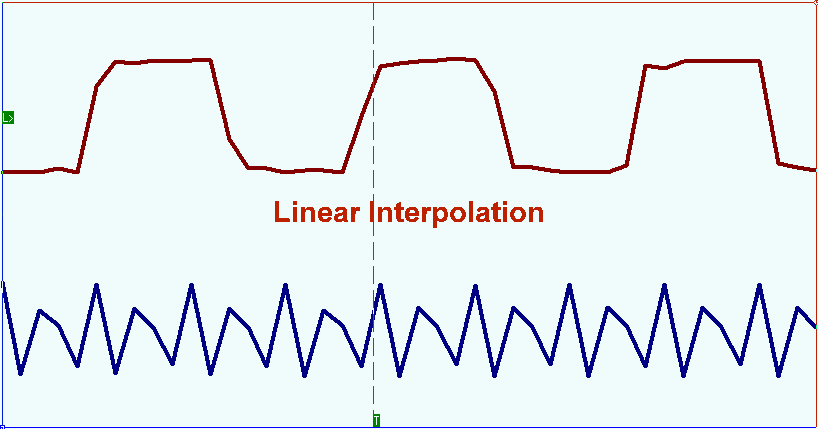For DSO this playback mode corresponds with the polyline detailed drawing style (Interpolation Off).

When DSO works in extreme situations, when the frequency of the registered signal is close to the half of the sample rate, the good result at the signal reconstruction is obtained by (Sin x)/x interpolation .

The picture shows the signal oscillogram with the (Sin x)/x interpolation  . As you can see the main signal harmonic is fully restored.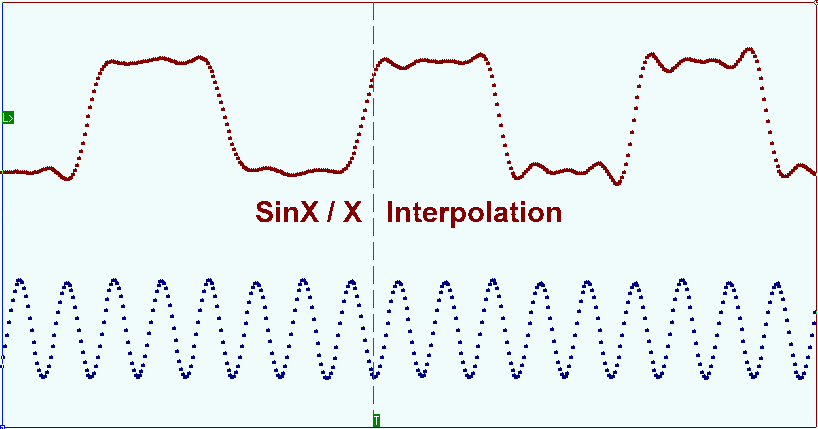In cases when the number of the samples under registration for the input signal frequency period is too high (dozens or more) spline interpolation can be used. When using this method the interpolation interval is divided into small segments and on each of them a function is set by the cubed polynomial. Polynomial ratios are chosen depending on some certain conditions. The general requirements for all types of tertiary splines are the function continuity and its passing through all points prescribed. Additional requirements can be function linearity between the points of the samples, higher derivatives continuity etc.

The picture below shows the pulse waveform oscillogram. Bright points show the samples, the solid line demonstrates the signal reconstruction result on these samples with the Bessel splines interpolation.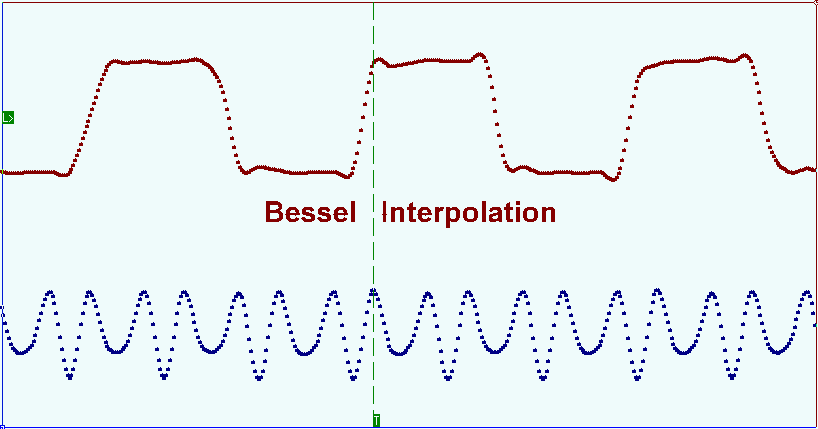Red circles mark the shoots appeared before the front edge and the falling edge of the displayed signal. Akima splines interpolation method allows minimizing the mentioned distortion. This method takes into account the initial function smoothness on the areas next to the sample points when choosing derivative in these points. The next picture shows the same signal oscillogram with the use of Akima splines interpolation.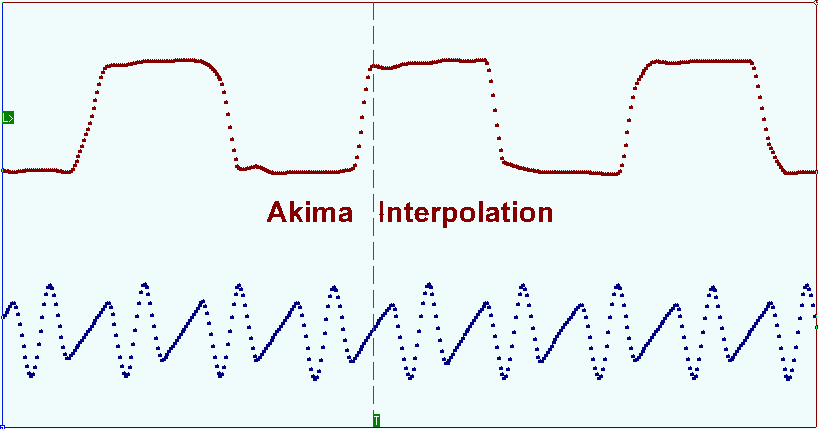Back to the list

Units Converter
• How it Works
• Company News
• Industry News & Events
• Measurement Fun Facts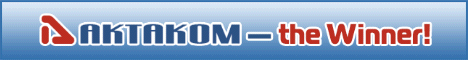Site map|Privacy policy|Terms of Use & Store Policies|How to Buy|Shipping|Payment|© T&M Atlantic, Inc., 2010-2022